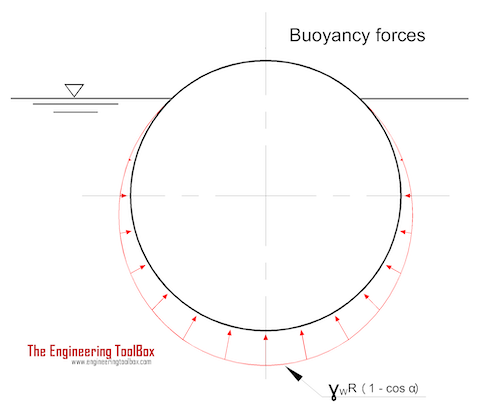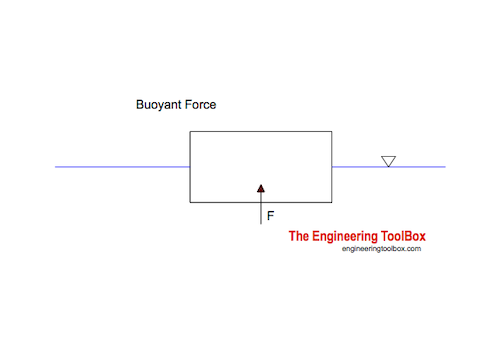Engineering ToolBox - Resources, Tools and Basic Information for Engineering and Design of Technical Applications!

# Buoyancy

## Buoyancy is the resultant force acting on a submerged body.Buoyancy is defined as the tendency of a body to float or rise when submerged in a fluid. The resultant force acting on a submerged body by the fluid is called the buoyant force and can be expressed as

F = V γ

= V ρ g                  (1)

where

F = buoyant force (N)

V = body volume (m3)

γ = ρ g = specific weight of fluid (N/m3)

ρ = density of fluid (kg/m3)

g = acceleration of gravity (= 9.81 m/s2)

The buoyant force acts upwards.

Archimedes' principle indicates that

"the upward buoyant force that is exerted on a body fully or partially submerged in a fluid - equals to the weight of the fluid that the body displaces"

• if the body weighs more than the fluid - it sinks
• if the body weighs less than the fluid - it floats

### Example - Buoyant Force acting on a Floating BoxA plastic box with length 0.3 m and width 0.4 m is submerged 0.1 m into water.  The water density is 1000 kg/m3.

The buoyant force acting on the box can be calculated with (1)

F = (0.3 m) (0.4 m) (0.1 m) (1000 kg/m3) (9.81 m/s2)

= 119 N

## Related Topics

• ### Fluid Mechanics

The study of fluids - liquids and gases. Involving velocity, pressure, density and temperature as functions of space and time.

## Related Documents

• ### Center of Gravity

A body and the center of gravity.
• ### Center of Gravity and Buoyancy

Stability - the center of gravity vs. the center of buoyancy.
• ### Equilibrant Force

The force required to keep a system of forces in equilibrium.
• ### Hot Air Balloons - Calculate Lifting Weights

Calculate hot air ballons lifting forces.
• ### Hydrostatic Force acting on Submerged Surface

Calculate the thrust force acting on a submerged surface.
• ### Hydrostatic Pressure vs. Depth

Depth and hydrostatic pressure.

## Engineering ToolBox - SketchUp Extension - Online 3D modeling!

Add standard and customized parametric components - like flange beams, lumbers, piping, stairs and more - to your Sketchup model with the Engineering ToolBox - SketchUp Extension - enabled for use with older versions of the amazing SketchUp Make and the newer "up to date" SketchUp Pro . Add the Engineering ToolBox extension to your SketchUp Make/Pro from the Extension Warehouse !

We don't collect information from our users. More about

## Citation

• The Engineering ToolBox (2009). Buoyancy. [online] Available at: https://www.engineeringtoolbox.com/buoyancy-force-d_1485.html [Accessed Day Month Year].

Modify the access date according your visit.

9.29.12